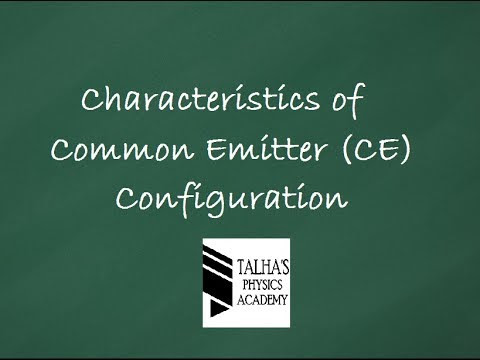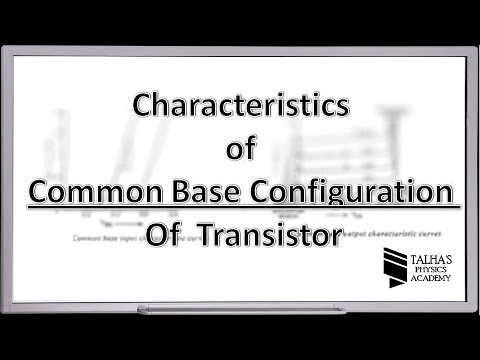## Posts

Showing posts from May, 2013

### What should be the lengths of an aluminium and a brass rods at STP.so that brass rod is longer than the aluminium rod by 4cm at all conditions of temperature.(alpha alumn 24exp -6 brass 20 expo -6c

Data :
Let Length of aluminum = La Length of brass = Lb =La + 0.04 (In meters) α for aluminum= 24x10 -6 c-1 α for brass= 20 x10 -6 c-1 Lengths at 0 0C La’ = ? and Lb’=?
Solution:
Length of aluminum is given by La’=La ( 1 + α Δt)----------------1 Lb’=Lb ( 1 + α Δt)-----------------2 Subtracting eq(1) from eq(2), we get La’ - Lb’ =La ( 1 + α Δt) - Lb ( 1 + α Δt) = La + La α Δt) - Lb + Lb α Δt =(La – Lb )+ Δt (La α - Lb α) 0.04 =0.04 + Δt (La α - Lb α) So Δt (La α - Lb α) =0 Since La α = Lb α La x 24x10 -6 = Lb x20 x10 -6 La =(20 /24) x Lb =20 Lb / 24 ------------3 Since Lb =La + 0.04 Putting value of La from eq (3) , we get Lb =(20 Lb / 24) +0.04or 24 x (Lb -0.04)=20 Lb 24 Lb – 20 Lb =0.96or Lb= 0.96/4 =Lb=0.24 m Answer Now La= 0.24 -0.04 =0.02 m Answer

### A x.ray photon of wavelength 1.82 exponent-10m is scattered at angle θ.if fractional change in wavelength is 2% then what is value of scattering angle?

A x.ray photon of wavelength 1.82 exponent-10m is scattered at angle θ.if fractional change in wavelength is 2% then what is value of scattering angle?
Data: λ = 1.82 x 10 -10 m Δ λ / λ = 2% θ = ?
Solution: According to Compton’s Shift we can write as Δ λ =(h / mo c) ( 1- Cos θ) ----------(1) Finding Δ λ Δ λ = 2 % x λ Δ λ = 2 /100 x 1.82 x 10 -10 =3.64 x 10 -12m Now using equation (1) 1- Cos θ = Δ λ x mo c /h or Cos θ = 1- (Δ λ x mo c /h) = 1 – (3.64 x 10 -12x 3 x 10 8 x 9.1 x 10 -31 / 6.63 x 10 -34)=-0.4988 Or θ=Cos-1 (-0.4988) =120 0 Answer

### COMMON EMITTER CONFIGURATION OF A TRANSISTORCOMMON EMITTER CONNECTION

In this configuration, the input is applied between the base and the emitter and the output is taken from the collector and the emitter. In this connection, the emitter is common to both the input and the output circuits as shown in Fig. In the common emitter configuration the input current is the base current IB and the output current is the collector current IC. The ratio of change in collector current to the change in base current at constant collector-emitter voltage is called base current amplification factor ( ).

COMMON EMITTER CIRCUIT

A test circuit for determining the static characteristic of an NPN transistor is shown in Fig In this circuit emitter is common to both input and output circuits. To measure the base and collector current milli ammeters are connected in series with the base and the output circuits. Voltmeters are connected across the input and the output circuits to measure VBE and VCE There are two potentiometers R1 and R2 to…

### COMMON BASE CONFIGURATION OF A TRANSISTORCOMMON BASE CONNECTION In this configuration the input is applied between the emitter and base and the output is taken from the collector and the base. Here the base is common to both the input and the output circuits as shown in Fig.

In a common base configuration, the input current is the emitter current. and the output current is the collector current I The ratio of change in collector

current to the change in emitter current at constant collector-base voltage is called current amplification factor,

In a transistor VEB, IE, VCB, and IC are parameters.

These parameters can be interrelated in a number of ways. In these parameters the input current and the output voltage are taken as independent variables.The input voltage and output current are then expressed in terms of these independent variables. And these dependent variables also be expressed in functional relationship.
i.e., VBE= f1 (IE,VCB)

…

### COMMON COLLECTOR CONFIGURATION OF A TRANSISTORCOMMON COLLECTOR CONNECTION

In  this  configuration  the  input  is  applied  between the  base  and  the  collector and  the  output  is  taken  from  the  collector  and  the  emitter.  Here  the  collector  is common to both the input and the output circuits as shown in Fig.

Common Collector Transistor Circuit

In  common  collector  configuration  the  input  current  is  the  base current  IB  and  the output current is the emitter current IE. The ratio of change in emitter current to the  change in the base current is called current amplification factor.

It is represented by

COMMON COLLECTOR CIRCUIT

A test  circuit  for determining the  static characteristic  of an NPN transistor is shown in Fig. In this circuit the collector is common to both the input and the output circuits.   To   measure   the   base   and   the   emitter   currents,   milli   ammeters   are connected in series with the base and the emitter circuits. Voltmeters are connected   across the input an…

### Q. The matrices representing the angular momentum components jx jy jz are all hermitian .Show that the Eigen values of j2 is equal TO J2 =JX2 +JY2+JZ2 are REAL AND NON-NEGATIVE.

Ans. This question is taken from the "Mathematical Methods for Physicist" Problem number 3.5 from chapter no 3

Finding the eigen values for J2=Jx2 + Jy2 + Jz2 Then we get (Jm |J2|Jm)= (Jm |Jx2|Jm) +(Jm |Jy2|Jm)+ (Jm |Jz2|Jm) Which can also be written as |Jx(jm)|2 + |Jy(jm)|2 + |Jz(jm)|2 The above equation shows that the eigen values for J2=Jx2 + Jy2 + Jz2 are real and non negative for complete understanding download and See Chapter 3 page no. 19